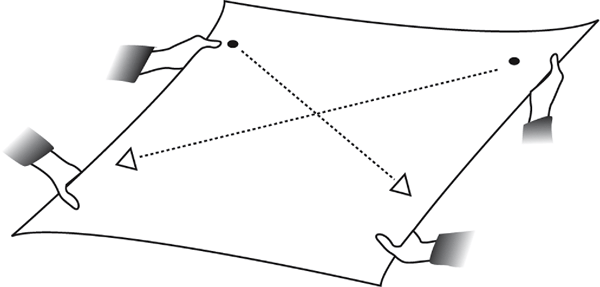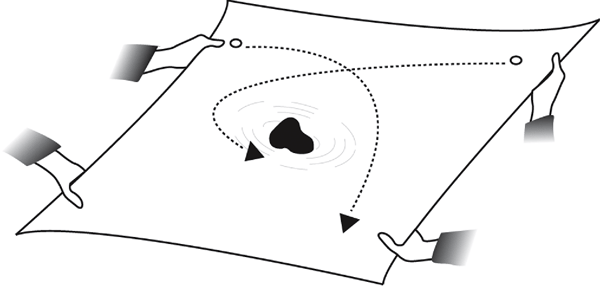# Gravity and Its Effect on Planetary Orbits

### 1. Introduction:

• Review the definition of gravity
• Drop a ball and explain why it falls downward
• Explain that the strength of a gravitational pull is determined by the masses of the objects involved and the distance between the objects
• Hold up a scale model of Earth and a scale model of the Sun. Inquire as to which object would exert a stronger pull
• ### 2. Materials:

• Large Rubber or plastic sheet
• Heavy round object, such as an 8-lb medicine ball or round watermelon
• Smaller, lighter objects, such as tennis balls
• ### 3. Activity:

• Explain that the sheet represents spacetime, the large object represents the Sun and the smaller object represents a planet
• Have the students pull the edges of the sheet outward. Make sure the sheet is taut.
• Roll the lighter balls across the sheet. Note how they travel in a straight line.
•• Place the heavy object in the center of the sheet. Keep the sheet pulled taut.
• Roll the lighter balls across the sheet once again. Note how they now curve. This illustrates Kepler's First Law that states a planet orbits the Sun in an elliptical orbit (Ideally there would be no friction and the lighter balls would make a complete elliptical orbit.)
•### 4. Closure:

• Discuss how large massive objects such as the Sun curve spacetime toward them (i.e., the ball follows a curved path)
• Discuss how smaller objects such as the ball, or planets, follow the curve of spacetime created by the more massive object
• ### 5. Extensions:

• Demonstrate or let the students demonstrate Kepler's Three Laws on an orbit simulator at http://observe.ivv.nasa.gov/nasa/education/reference/orbits/orbit1.html
• Demonstrate different types of orbit by manipulating data at http://www.fourmilab.ch/cgi-bin/uncgi/Solar

Credit:

Artwork by Aurore Simmonet

This activity is part of the StarChild site.

http://starchild.gsfc.nasa.gov/